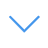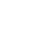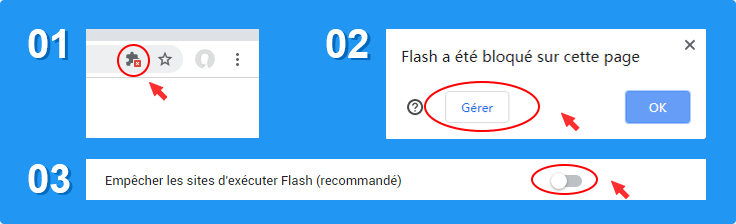# Solve Math

Solve Math is one of the Math Games that you can play on UGameZone.com for free. Are you sensitive to mathematical calculations? This game challenges the player's responsiveness and mathematical computing skills. You have to solve the equals answer by given random numbers and mathematical symbols.

Read MoreAppuyez sur Echap pour quitter le plein écran.

# Solve Math

Solve Math is one of the Math Games that you can play on UGameZone.com for free. Are you sensitive to mathematical calculations? This game challenges the player's responsiveness and mathematical computing skills. You have to solve the equals answer by given random numbers and mathematical symbols.Pour jouer au jeu, vous devez autoriser Adobe Flash Player à fonctionner sur votre navigateur.

Vous pouvez utiliser le plugin UGameZone Flash Enabler pour activer Flash automatiquement. Ou vous pouvez suivre vos instructions pour activer Flash manuellement.

Jouer automatiquement

## Game Ratings

75%

Pièces 500

Évaluation 75%

700
0
Category
Math Games
Mots clés
La description

Solve Math is one of the Math Games that you can play on UGameZone.com for free. Are you sensitive to mathematical calculations? This game challenges the player's responsiveness and mathematical computing skills. You have to solve the equals answer by given random numbers and mathematical symbols.

Étape 1
Étape 2
Veuillez activer Adobe Flash Player pour jouer à "Solve Math"

Activer les paramètres de Flash dans Chrome: uis cliquez sur " Empêcher les sites d'exécuter Flash . Activez l'option " Demander d'abord ". Comme ci-dessous:Cliquez maintenant sur et cliquez sur le bouton " Autoriser " dans Chrome, en haut à gauche, pour jouer au jeu. Comme ci-dessous: